There are 366 different Starters of The Day, many to choose from. You will find below some starters on the topic of Puzzles. A lesson starter does not have to be on the same topic as the main part of the lesson or the topic of the previous lesson. It is often very useful to revise or explore other concepts by using a starter based on a totally different area of Mathematics.

Main Page

Puzzles Starters: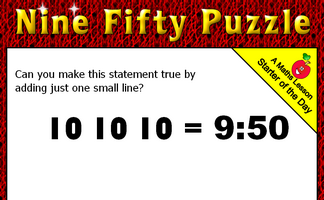A little lateral thinking might help you solve this puzzle.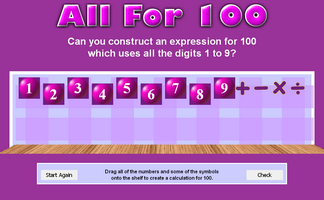Can you write an expression for 100 which uses all the digits 1 to 9?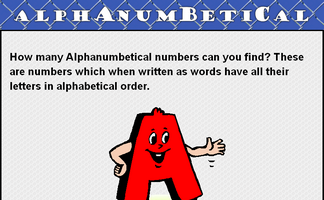A challenge to find the number which when written as a word has all the letters in alphabetical order.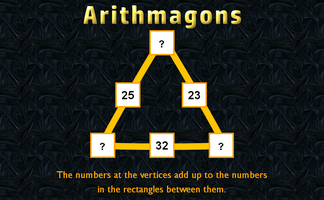This lesson starter requires pupils to find the missing numbers in this partly completed arithmagon puzzle.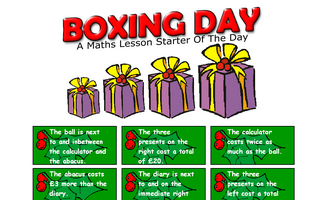Work out the contents and the cost of the Christmas boxes from the given clues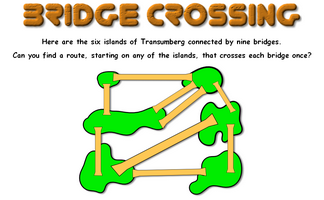The classic puzzle of finding a route which crosses each bridge once.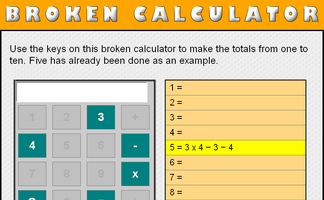Some keys are missing from this calculator. Just how useful is it?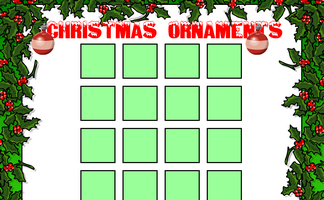A Maths Starter activity requiring students to arrange Christmas ornaments in a square box.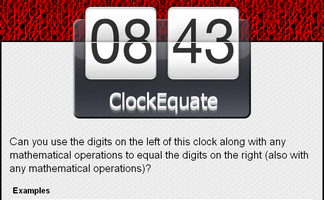Can you use the digits on the left of this clock along with any mathematical operations to equal the digits on the right?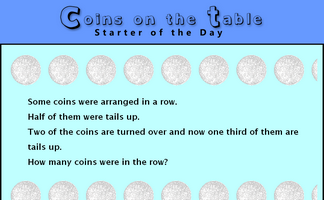A puzzle about the number of coins on a table given information about fractions of them.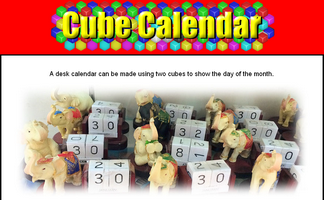What numbers should be on each face of the two cubes to make this perpetual calendar?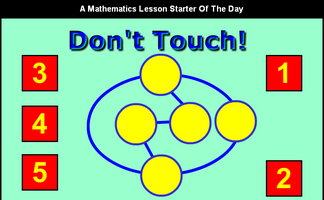A number placing puzzle which, when solved, raises the notion of proof.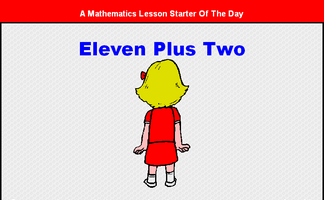Rearrange the letters in the expression 'Eleven Plus Two' to make another expression with the same value.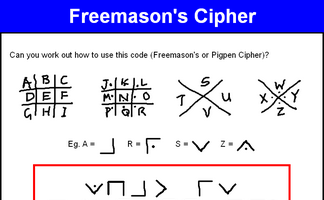Find symmetric words in this ancient cipher.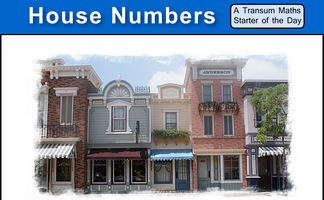The numbers on five houses next to each other add up to 70. What are those five numbers?A little lateral thinking will help you solve this number puzzle.Work out how many squares are there altogether in the given pattern then tackle the chess board.How many squares can be found by joining four dots on the grid?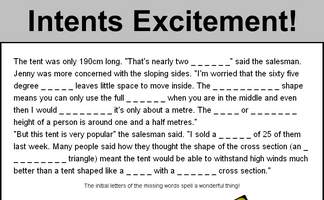Work out the mathematical words missing from the story about buying a tent.Change the numbers on the apples so that the number on the lemon is the given total.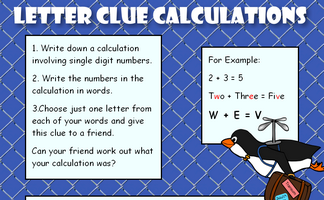Work out what the calculations might be from the letter clues.Match the fractions to their decimal equivalents and join them with lines that do not cross.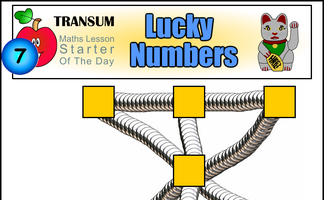Arrange the numbers so that the totals of the three numbers along any line are the same.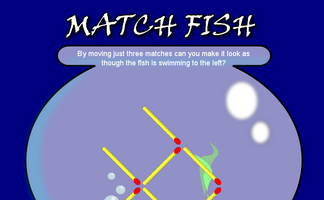A classic matchstick puzzle designed to challenge your spacial awareness.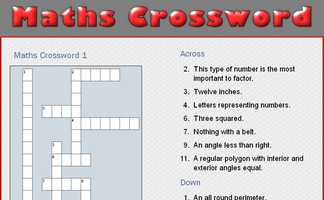A small crossword puzzle containing mathematical words.Find mathematical words which can be made by using the letters in the name of your school?Guess the four digit number with help from the clues.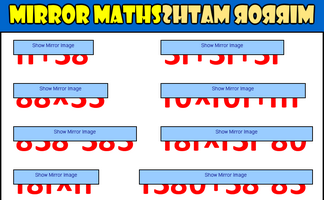The bottom half of some symmetrical calculations are shown above. Can you work out the answers?A puzzle about a restaurant bill. Exactly where did the missing pound go?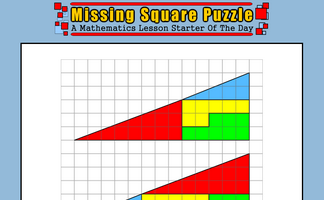The missing square puzzle is an optical illusion used to help students reason about geometrical figures.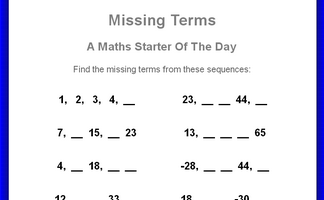Find the missing terms from these linear sequences.Work out which team played which from the information given.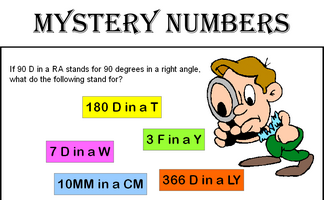Can you recognise the mystery numbers from the clues?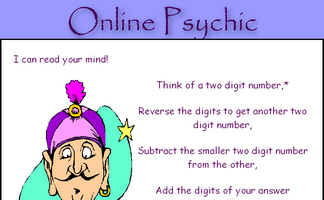Work out why subtracting a two digit number from its reverse gives a multiple of nine.Follow the compass directions given in the squares to find where the route starts?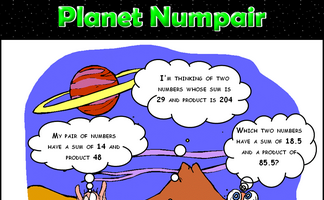Arrange the digits one to nine to make a correct addition calculation.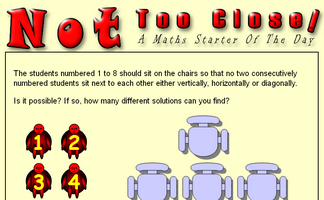This activity requires eight students to sit non consecutively on a grid of chairs.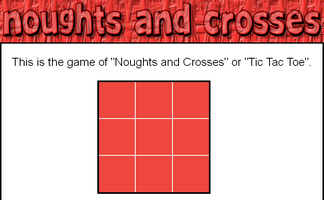In how many different ways can the first X and O by placed on the grid?How many ways can you write an expression for 100 which only uses the same digit repeated and any operations?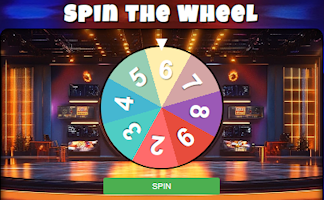Work out the least amount of time for four people to walk through a tunnel?Can you solve this paradox by agreeing whether the statements are right or wrong?Five numbers are added together in pairs and the sums shown. What might the five numbers be?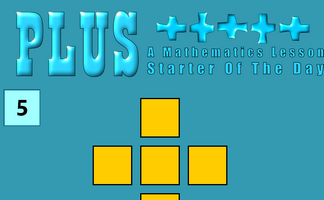A number puzzle suitable for children with a wide range of abilities.Go around the roundabout performing each of the operations. Which starting point gives the largest answer?Work out the cheapest way from Los Angeles to Las Vegas by choosing the best route and adding up the given numbers.Can you draw 4 straight lines, without taking your pencil off the paper, which pass through all 9 roses?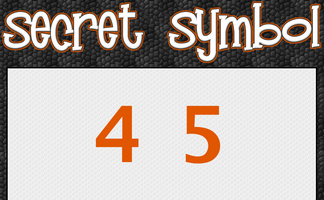Place a symbol between the four and the five to get a number greater than four and less than five.Can you find a 14 digit number containing two each of the digits one to seven which obeys the rules given?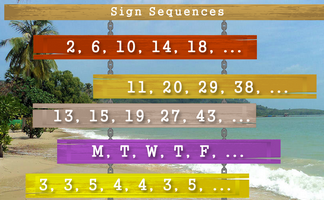Continue the sequences if you can work out the rule.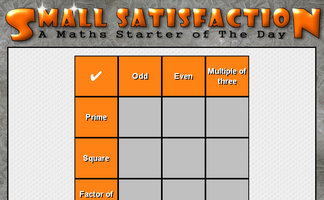Arrange the digits one to nine in the grid so that they obey the row and column headings.Arrange the numbered trees so that adjacent sums are square numbers.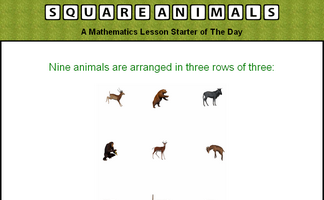Separate three rows of three animals using three squares.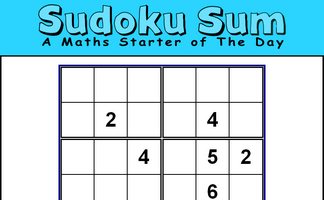What is the sum of the numbers missing from the given Sudoku puzzle?Interactive number-based logic puzzle similar to those featuring in The Times and Telegraph newspapers.Each traffic sign stands for a number. Some of the sums of rows and columns are shown. What numbers might the signs stand for?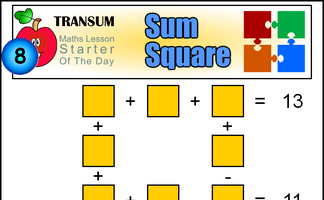Arrange the numbers one to eight into the calculations to make the totals correct..THOAN stands for 'Think of a number' and there are four randomly generated THOAN puzzles to solve.Place the numbers on the triangle so that the totals along each of the sides are equal.A tricky problem set on a coordinate grid.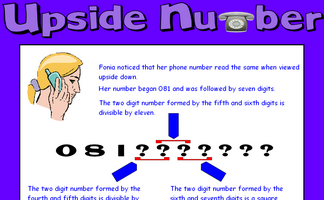Work out the phone number from the clues given.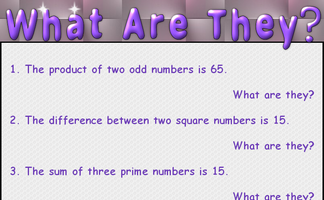A starter about sums, products, differences, ratios, square and prime numbers.Create your own mathematical word search for the whole class to enjoy.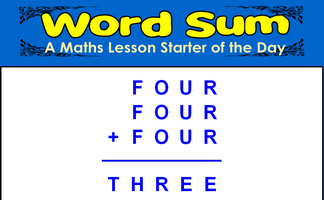Each letter stands for a different digit. Can you make sense of word sum?

Search

The activity you are looking for may have been classified in a different way from the way you were expecting. You can search the whole of Transum Maths by using the box below.

Have today's Starter of the Day as your default homepage. Copy the URL below then select
Tools > Internet Options (Internet Explorer) then paste the URL into the homepage field.

Set as your homepage (if you are using Internet Explorer)

Do you have any comments? It is always useful to receive feedback and helps make this free resource even more useful for those learning Mathematics anywhere in the world. Click here to enter your comments.For All: